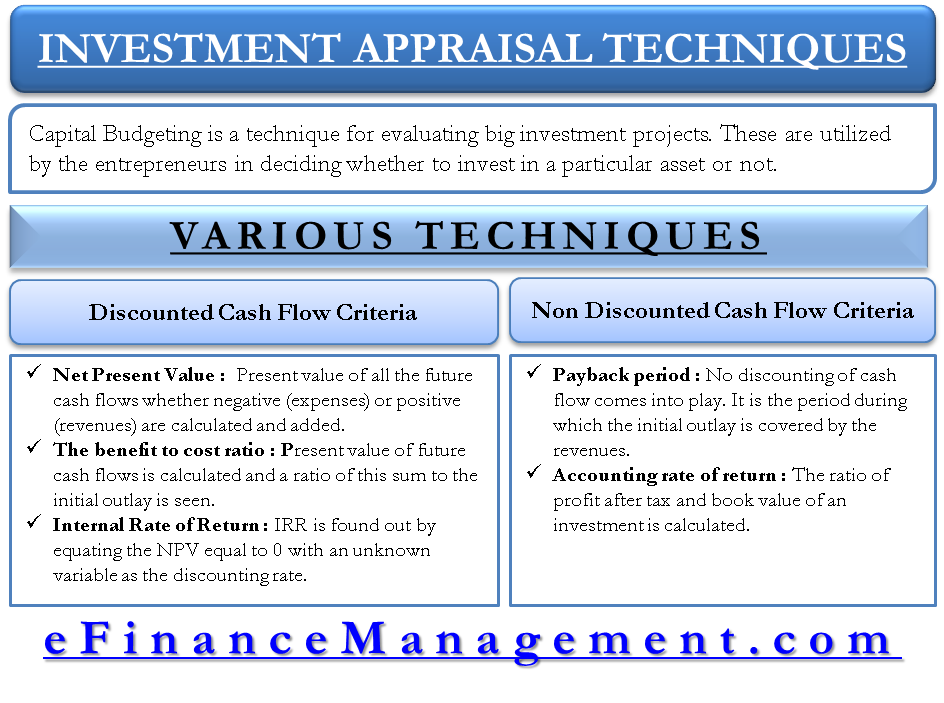# Capital Budgeting – 5 Investment Appraisal Techniques

Capital budgeting is a technique for evaluating big investment projects. Net Present Value (NPV), Benefit to Cost Ratio, Internal Rate of Return (IRR), Payback Period and Accounting Rate of Return are some prominent capital budgeting techniques widely used in the finance arena. A project is passed for implementation after it is approved by these techniques.

Capital budgeting techniques are utilized by the entrepreneurs in deciding whether to invest in a particular asset or not. It has to be performed very carefully because a huge sum of money is invested in fixed assets such as machinery, plant etc. The analysis is based on 2 things viz first, the stream of expected cash flows generated by utilizing the assets and second, initial or future outlays expected for acquiring the asset. Such investment appraisal tools or capital budgeting techniques are broadly classified into two criteria:

Discounted Cash Flow Criteria:

Discounted cash flow criteria have three techniques for evaluating an investment.

## Net Present Value (NPV)Capital Budgeting

Net present value (NPV) technique is a well-known method for evaluating investment projects or proposals. In this technique or method, the present value of all the future cash flows whether negative (expenses) or positive (revenues) are calculated using an appropriate discounting rate and added. From this sum, the initial outlay is deducted to find out the profit in present terms. If the figure is positive, the techniques show a green signal to the project and vice versa. This figure is called the net present value (NPV).

Suppose, our proposed investment is \$100 million and present value (PV) of future cash flows come to be \$120 million. The NPV would be \$20 million and hence, the project should be undertaken. If the PV is \$80 million, the NPV would be negative \$20, the project is not advisable in this case.

## The Benefit to Cost Ratio

The benefit to cost ratio presents the analysis in a proportion or ratio format. Here, just like in the NPV method, the present value of future cash flows is calculated and a ratio of this sum to the initial outlay is seen. If this ratio is more than 1, the project should be accepted and if it is less than 1, it should be rejected.

Let us assume the same example as taken in the NPV method. The benefit to cost ratio would be 1.2 in the first case and as per the rule, the project should be executed and in the second example, the ratio is 0.8 which is less than 1, so the project should be rejected.

## Internal Rate of Return

This method is also a well-known method of evaluation. This has a severe connection with the first method i.e. Net Present Value (NPV). In the NPV method, the discounting rate is assumed to have known to the evaluator. On the contrary, the rate of discounting is not known in this method of Internal Rate of Return (IRR). IRR is found out by equating the NPV equal to 0 with an unknown variable as the discounting rate. This discounting rate is found out using trial and error method or extrapolating and interpolating methods and it is known as Internal Rate of Return (IRR). For evaluation purpose, IRR is compared with the cost of capital of the organization. If the IRR is greater than a cost of capital, the project should be accepted and vice versa.Non-Discounting Cash Flow Criteria:

Non-discounting cash flow criteria have 2 techniques for appraisal of such investments.

## Payback Period (PBP)

Payback period is the method of evaluation where no discounting of cash flow comes into play. The term ‘payback period’ is the period during which the initial outlay is covered by the revenues. Suppose an initial outlay is \$100 million and the revenue stream is \$40 for the first 4 years. Then, the payback period is 2.5 years. Essentially, in 2.5 years, the entrepreneur gets his investment back and revenue after this period is the profit for him.

## Accounting Rate of Return

Accounting rate of return is calculated with the help of accounting data. The ratio of profit after tax and book value of an investment is the accounting rate of return. If the book value of an investment is \$100 and profit after tax is \$25, then the ratio results into 25%. Hence, the accounting rate of return is 25%.

Last updated on : March 23rd, 2019
1.Habiba Akter
2.Sonam